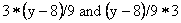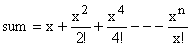Time: 3 Hours                                                                                                      Max. Marks: 100

NOTE: There are 9 Questions in all.

·      Question 1 is compulsory and carries 20 marks. Answer to Q. 1. must be written in the space provided for it in the answer book supplied and nowhere else.

·      Out of the remaining EIGHT Questions answer any FIVE Questions. Each question carries 16 marks.

·      Any required data not explicitly given, may be suitably assumed and stated.

Q.1       Choose the correct or best alternative in the following:                       (2x10)

a.  If an integer needs two bytes of storage, then the maximum value of a signed integer is

(A)  216-1                                             (B)  215-1

(C)  216                                                (D)  215

b.      Literal means

(A)    a string                                         (B)  a string constant

(C)  a character                                   (D)  an alphabet

c.   If ‘y’ is of integer type then the expressions(A)    must yield the same value.

(B)    must yield different values.

(C)    may or may not yield the same value.

(D)   none of the above.

d.   In the following code fragment

int x, y = 2, z, a;

x=(y*=2) + (z=a=y);

printf (‘%d’,x);

(A)  prints 8

(B)  prints 6

(C) prints 6 or 8 depending on the compiler

(D)  is syntactically wrong

e.   A possible output of the following program fragment is

for (i=getchar();; i=get.char())

if (i==‘x’) break;

else putchar(i);

(A)     mi                                                (B)  mix

(C)  mixx                                             (D)  none of the above

f.    In a for loop, if the condition is missing, then,

(A)     It is assumed to be present and taken to be false.

(B)     It is assumed to be present and taken to be true.

(C)     It results in a syntax error.

(D)  Execution will be terminated abruptly.

g.   If storage class is missing in the array definition, by default it will be taken to be

(A)     automatic

(B)     external

(C)     static

(D)    either automatic or external depending on the place of occurrence.

h.   The maximum number of dimensions an array can have in C is

(A)    3                                                  (B) 4

(C) 5                                                   (D) compiler dependent

i.    puts(argv);

(A)   prints the name of the source code file.

(B)   prints argv.

(C) prints the number of command line arguments.

(D) prints the name of the executable code file.

j.    Consider the following declaration:

int a, *b=&a, **c = &b;

The following program fragment

a = 4;

**c=5;

(A)  does not change the value of a      (B)  assigns address of c to a

(C)  assigns  5 to a                               (D)  assigns the value of b to a

Answer any FIVE Questions out of EIGHT Questions.

Each question carries 16 marks.

Q.2     a.   Explain top-down concept of  program design.                                                   (4)

b.   What is a compiler? What type of errors can be detected by it? How does it differ from an interpreter?                                                                  (2+3+3)

c.   What is a subroutine? How do subroutines help in program writing?                (2+2)

Q.3     a.   Define the term ‘complexity of an algorithm; and explain worst-case and average case analysis of an algorithm.                                            (2+4)

b.   Write an algorithm to reverse the digits of an integer.                                           (4)

c.   Write a C program to generate a series of Armstrong numbers. A number is an Armstrong number if the sum of the cube of its digit is equal to the number.                                                                      (6)

Q.4     a.   Write a C program to generate the following series:(8)

b.   What is bubble sort? Write a program to sort a list of n elements of an array using bubble sort.                                                                   (8)

Q.5     a.   Explain the difference between a function declaration and function definition.                       (4)

b.   What is a pre-processor? Which pre-processor is used to define a macro?         (4)

c.   What are the different storage classes in C?                                                        (4)

d.   Differentiate between recursion and iteration.                                                      (4)

Q.6     a.   Differentiate between pointer (*) and address (&) operator using examples.        (4)

b.   Write a program to find the highest of three numbers using pointer to function.                     (6)

c.       Differentiate between

i.      Static variables and auto variables.

ii.      Execution error and compilation error.                                        (6)

Q.7     a.   Explain the use of structures with pointers.                                                          (5)

b.   Can a structure be used within a structure? Give appropriate examples to support your answer.                                                                 (6)

c.   What are the different modes in which a file can be opened?                               (5)

Q.8     a.   Write a C program to insert a new node at a specified position in a link list.       (8)

b.   Distinguish between the functions islower() and tolower().                                   (2)

c.   Write a C program that reads two strings and copies the smaller string into the bigger string.                                                                       (6)

Q.9     a.   Explain black box testing.                                                                                   (4)

b.   Why is C standard library needed?                                                                     (4)

c.   What are the functions of the following header files:

(i)                  ctype.h

(ii)                string.h                                                                                                  (4)

d.  Differentiate between call by reference and call by value.                                      (4)